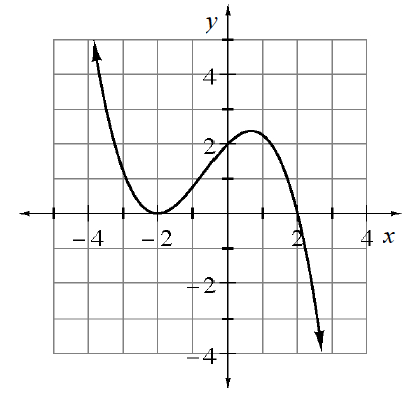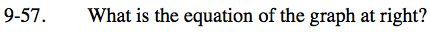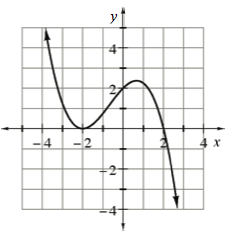### Home > INT3 > Chapter Ch9 > Lesson 9.1.4 > Problem9-57

9-57.

What is the equation of the graph below? 9-57 HW eTool (Desmos). Homework Help ✎Write the equation by using the x-intercepts. Don't forget you have a double root at x = −2. Add your constant of proportionality.

$y=k(x+2)(x+2)(x-2)$

Find the constant of proportionality by substituting the y-intercept (0, 2) for x and y.

$y =-\frac{1}{4}(x-2)(x+2)^2$

Use the eTool below to help you with this problem.
Click the link at right for the full version of the eTool: INT3 9-57 HW eTool.